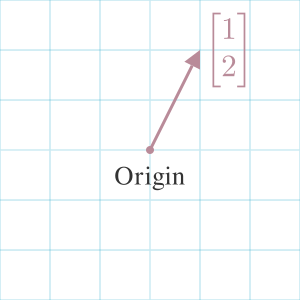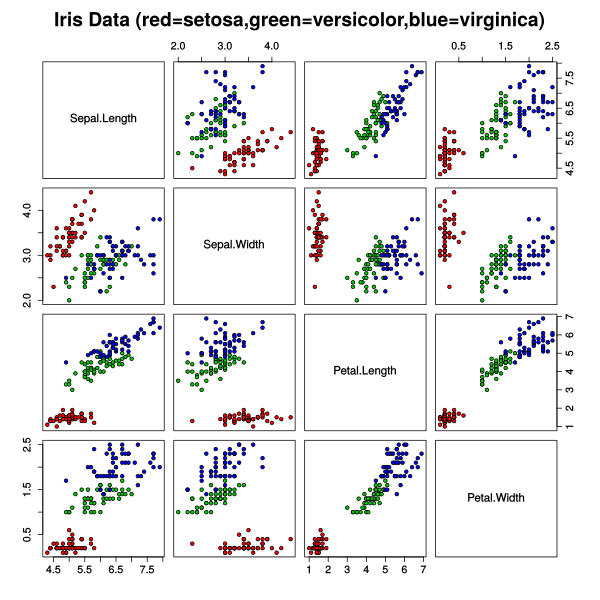## VectorFor most practical purposes, a vector is an ordered list of numbers.

In linear algebra, the numbers describe an arrow pointing in space. If we create a grid to represent space, we will place the tip at the coordinate in the vector and the base on the origin of the grid system. In 2d space, that origin is typically the point (0, 0).

We can think of the vectors describing a unit of change along each axis. For example, the vector in the cover image is $(1, 2)$ or 1 unit of "change" along the x-axis and two units of "change" along the y-axis. That's why we commonly describe vectors as being defined by a length (or magnitude) and direction.

We refer to each number in a vector as a component.

Vectors are represented in math as a vertical list of numbers, or a matrix with one column, to differential from coordinates.

$\vec{A} = \begin{bmatrix}1\\2\end{bmatrix}$

We can use Pythagoras Theorem to calculate the length of a vector by squaring each vector component, then taking the square root of their sum:

$\| \vec{A} \| = \sqrt{1^2 + 2^2}$

A computer scientist may think of vectors simply as an array. We can use vectors to represent a model of something. For example, the Iris Flower Dataset represents flowers as a vector of 4 attributes:

$\begin{bmatrix} \text{sepal length}\\ \text{sepal width}\\ \text{petal length in cm}\\ \text{petal width in cm} \end{bmatrix}$

We can plot these numbers in a coordinate system to see how the flowers relate to each other. This example from Wikipedia generates a 2d scatterplot for each pair of values.We describe a Ray with a similar notation to vectors. However, a Ray doesn't have a length. Only a direction to continue infinitely.

3Blue1Brown (2016)

#### References

3Blue1Brown. Vectors | Chapter 1, Essence of linear algebra. August 2016. URL: https://www.youtube.com/watch?v=fNk_zzaMoSs.